# ML Interview Questions

## Question Set 1

#### Machine Learning Interview Questions: 4 Categories

We’ve traditionally seen machine learning interview questions pop up in several categories.
1. 1.
The first really has to do with the algorithms and theory behind machine learning. You’ll have to show an understanding of how algorithms compare with one another and how to measure their efficacy and accuracy in the right way.
2. 2.
The second category has to do with your programming skills and your ability to execute on top of those algorithms and the theory.
3. 3.
The third has to do with your general interest in machine learning. You’ll be asked about what’s going on in the industry and how you keep up with the latest machine learning trends.
4. 4.
Finally, there are company or industry-specific questions that test your ability to take your general machine learning knowledge and turn it into actionable points to drive the bottom line forward.
We’ve divided this guide to machine learning interview questions into the categories we mentioned above so that you can more easily get to the information you need when it comes to machine learning interview questions.

#### Machine Learning Interview Questions: Algorithms/Theory

These algorithms questions will test your grasp of the theory behind machine learning.
Q1: What’s the trade-off between bias and variance?
Answer: Bias is error due to erroneous or overly simplistic assumptions in the learning algorithm you’re using. This can lead to the model underfitting your data, making it hard for it to have high predictive accuracy and for you to generalize your knowledge from the training set to the test set.
Variance is error due to too much complexity in the learning algorithm you’re using. This leads to the algorithm being highly sensitive to high degrees of variation in your training data, which can lead your model to overfit the data. You’ll be carrying too much noise from your training data for your model to be very useful for your test data.
The bias-variance decomposition essentially decomposes the learning error from any algorithm by adding the bias, the variance and a bit of irreducible error due to noise in the underlying dataset. Essentially, if you make the model more complex and add more variables, you’ll lose bias but gain some variance — in order to get the optimally reduced amount of error, you’ll have to tradeoff bias and variance. You don’t want either high bias or high variance in your model.
Q2: What is the difference between supervised and unsupervised machine learning?
Answer: Supervised learning requires training labeled data. For example, in order to do classification (a supervised learning task), you’ll need to first label the data you’ll use to train the model to classify data into your labeled groups. Unsupervised learning, in contrast, does not require labeling data explicitly.
Q3: How is KNN different from k-means clustering?
Answer: K-Nearest Neighbors is a supervised classification algorithm, while k-means clustering is an unsupervised clustering algorithm. While the mechanisms may seem similar at first, what this really means is that in order for K-Nearest Neighbors to work, you need labeled data you want to classify an unlabeled point into (thus the nearest neighbor part). K-means clustering requires only a set of unlabeled points and a threshold: the algorithm will take unlabeled points and gradually learn how to cluster them into groups by computing the mean of the distance between different points.
The critical difference here is that KNN needs labeled points and is thus supervised learning, while k-means doesn’t—and is thus unsupervised learning.
Q4: Explain how a ROC curve works.
Answer: The ROC curve is a graphical representation of the contrast between true positive rates and the false positive rate at various thresholds. It’s often used as a proxy for the trade-off between the sensitivity of the model (true positives) vs the fall-out or the probability it will trigger a false alarm (false positives).
Q5: Define precision and recall.
Answer: Recall is also known as the true positive rate: the amount of positives your model claims compared to the actual number of positives there are throughout the data. Precision is also known as the positive predictive value, and it is a measure of the amount of accurate positives your model claims compared to the number of positives it actually claims. It can be easier to think of recall and precision in the context of a case where you’ve predicted that there were 10 apples and 5 oranges in a case of 10 apples. You’d have perfect recall (there are actually 10 apples, and you predicted there would be 10) but 66.7% precision because out of the 15 events you predicted, only 10 (the apples) are correct.
Q6: What is Bayes’ Theorem? How is it useful in a machine learning context?
Answer: Bayes’ Theorem gives you the posterior probability of an event given what is known as prior knowledge.
Mathematically, it’s expressed as the true positive rate of a condition sample divided by the sum of the false positive rate of the population and the true positive rate of a condition. Say you had a 60% chance of actually having the flu after a flu test, but out of people who had the flu, the test will be false 50% of the time, and the overall population only has a 5% chance of having the flu. Would you actually have a 60% chance of having the flu after having a positive test?
Bayes’ Theorem says no. It says that you have a (.6 * 0.05) (True Positive Rate of a Condition Sample) / (.6*0.05)(True Positive Rate of a Condition Sample) + (.5*0.95) (False Positive Rate of a Population) = 0.0594 or 5.94% chance of getting a flu.
Bayes’ Theorem is the basis behind a branch of machine learning that most notably includes the Naive Bayes classifier. That’s something important to consider when you’re faced with machine learning interview questions.
Q7: Why is “Naive” Bayes naive?
Answer: Despite its practical applications, especially in text mining, Naive Bayes is considered “Naive” because it makes an assumption that is virtually impossible to see in real-life data: the conditional probability is calculated as the pure product of the individual probabilities of components. This implies the absolute independence of features — a condition probably never met in real life.
As a Quora commenter put it whimsically, a Naive Bayes classifier that figured out that you liked pickles and ice cream would probably naively recommend you a pickle ice cream.
Q8: Explain the difference between L1 and L2 regularization.
Answer: L2 regularization tends to spread error among all the terms, while L1 is more binary/sparse, with many variables either being assigned a 1 or 0 in weighting. L1 corresponds to setting a Laplacean prior on the terms, while L2 corresponds to a Gaussian prior.
Q9: What’s your favorite algorithm, and can you explain it to me in less than a minute?
Answer: This type of question tests your understanding of how to communicate complex and technical nuances with poise and the ability to summarize quickly and efficiently. Make sure you have a choice and make sure you can explain different algorithms so simply and effectively that a five-year-old could grasp the basics!
Q10: What’s the difference between Type I and Type II error?
Answer: Don’t think that this is a trick question! Many machine learning interview questions will be an attempt to lob basic questions at you just to make sure you’re on top of your game and you’ve prepared all of your bases.
Type I error is a false positive, while Type II error is a false negative. Briefly stated, Type I error means claiming something has happened when it hasn’t, while Type II error means that you claim nothing is happening when in fact something is.
A clever way to think about this is to think of Type I error as telling a man he is pregnant, while Type II error means you tell a pregnant woman she isn’t carrying a baby.
Q11: What’s a Fourier transform?
Answer: A Fourier transform is a generic method to decompose generic functions into a superposition of symmetric functions. Or as this more intuitive tutorial puts it, given a smoothie, it’s how we find the recipe. The Fourier transform finds the set of cycle speeds, amplitudes, and phases to match any time signal. A Fourier transform converts a signal from time to frequency domain—it’s a very common way to extract features from audio signals or other time series such as sensor data.
Q12: What’s the difference between probability and likelihood?
TBD
Q13: What is deep learning, and how does it contrast with other machine learning algorithms?
Answer: Deep learning is a subset of machine learning that is concerned with neural networks: how to use backpropagation and certain principles from neuroscience to more accurately model large sets of unlabelled or semi-structured data. In that sense, deep learning represents an unsupervised learning algorithm that learns representations of data through the use of neural nets.
Q14: What’s the difference between a generative and discriminative model?
Answer: A generative model will learn categories of data while a discriminative model will simply learn the distinction between different categories of data. Discriminative models will generally outperform generative models on classification tasks.
Q15: What cross-validation technique would you use on a time series dataset?
Answer: Instead of using standard k-folds cross-validation, you have to pay attention to the fact that a time series is not randomly distributed data—it is inherently ordered by chronological order. If a pattern emerges in later time periods, for example, your model may still pick up on it even if that effect doesn’t hold in earlier years!
You’ll want to do something like forward chaining where you’ll be able to model on past data then look at forward-facing data.
• Fold 1 : training , test 
• Fold 2 : training [1 2], test 
• Fold 3 : training [1 2 3], test 
• Fold 4 : training [1 2 3 4], test 
• Fold 5 : training [1 2 3 4 5], test 
Q16: How is a decision tree pruned?
Answer: Pruning is what happens in decision trees when branches that have weak predictive power are removed in order to reduce the complexity of the model and increase the predictive accuracy of a decision tree model. Pruning can happen bottom-up and top-down, with approaches such as reduced error pruning and cost complexity pruning.
Reduced error pruning is perhaps the simplest version: replace each node. If it doesn’t decrease predictive accuracy, keep it pruned. While simple, this heuristic actually comes pretty close to an approach that would optimize for maximum accuracy.
Q17: Which is more important to you: model accuracy or model performance?
Answer: This question tests your grasp of the nuances of machine learning model performance! Machine learning interview questions often look towards the details. There are models with higher accuracy that can perform worse in predictive power—how does that make sense?
Well, it has everything to do with how model accuracy is only a subset of model performance, and at that, a sometimes misleading one. For example, if you wanted to detect fraud in a massive dataset with a sample of millions, a more accurate model would most likely predict no fraud at all if only a vast minority of cases were fraud. However, this would be useless for a predictive model—a model designed to find fraud that asserted there was no fraud at all! Questions like this help you demonstrate that you understand model accuracy isn’t the be-all and end-all of model performance.
Q18: What’s the F1 score? How would you use it?
Answer: The F1 score is a measure of a model’s performance. It is a weighted average of the precision and recall of a model, with results tending to 1 being the best, and those tending to 0 being the worst. You would use it in classification tests where true negatives don’t matter much.
Q19: How would you handle an imbalanced dataset?
Answer: An imbalanced dataset is when you have, for example, a classification test and 90% of the data is in one class. That leads to problems: an accuracy of 90% can be skewed if you have no predictive power on the other category of data! Here are a few tactics to get over the hump:
1. 1.
Collect more data to even the imbalances in the dataset.
2. 2.
Resample the dataset to correct for imbalances.
3. 3.
Try a different algorithm altogether on your dataset.
What’s important here is that you have a keen sense for what damage an unbalanced dataset can cause, and how to balance that.
Q20: When should you use classification over regression?
Answer: Classification produces discrete values and dataset to strict categories, while regression gives you continuous results that allow you to better distinguish differences between individual points. You would use classification over regression if you wanted your results to reflect the belongingness of data points in your dataset to certain explicit categories (ex: If you wanted to know whether a name was male or female rather than just how correlated they were with male and female names.)
Q21: Name an example where ensemble techniques might be useful.
Answer: Ensemble techniques use a combination of learning algorithms to optimize better predictive performance. They typically reduce overfitting in models and make the model more robust (unlikely to be influenced by small changes in the training data).
You could list some examples of ensemble methods (bagging, boosting, the “bucket of models” method) and demonstrate how they could increase predictive power.
Q22: How do you ensure you’re not overfitting with a model?
Answer: This is a simple restatement of a fundamental problem in machine learning: the possibility of overfitting training data and carrying the noise of that data through to the test set, thereby providing inaccurate generalizations.
There are three main methods to avoid overfitting:
1. 1.
Keep the model simpler: reduce variance by taking into account fewer variables and parameters, thereby removing some of the noise in the training data.
2. 2.
Use cross-validation techniques such as k-folds cross-validation.
3. 3.
Use regularization techniques such as LASSO that penalize certain model parameters if they’re likely to cause overfitting.
Q23: What evaluation approaches would you work to gauge the effectiveness of a machine learning model?
Answer: You would first split the dataset into training and test sets, or perhaps use cross-validation techniques to further segment the dataset into composite sets of training and test sets within the data. You should then implement a choice selection of performance metrics: here is a fairly comprehensive list. You could use measures such as the F1 score, the accuracy, and the confusion matrix. What’s important here is to demonstrate that you understand the nuances of how a model is measured and how to choose the right performance measures for the right situations.
Q24: How would you evaluate a logistic regression model?
Answer: A subsection of the question above. You have to demonstrate an understanding of what the typical goals of a logistic regression are (classification, prediction, etc.) and bring up a few examples and use cases.
Q25: What’s the “kernel trick” and how is it useful?
Answer: The Kernel trick involves kernel functions that can enable in higher-dimension spaces without explicitly calculating the coordinates of points within that dimension: instead, kernel functions compute the inner products between the images of all pairs of data in a feature space. This allows them the very useful attribute of calculating the coordinates of higher dimensions while being computationally cheaper than the explicit calculation of said coordinates. Many algorithms can be expressed in terms of inner products. Using the kernel trick enables us effectively run algorithms in a high-dimensional space with lower-dimensional data.

#### Machine Learning Interview Questions: Programming

These machine learning interview questions test your knowledge of programming principles you need to implement machine learning principles in practice. Machine learning interview questions tend to be technical questions that test your logic and programming skills: this section focuses more on the latter.
Q26: How do you handle missing or corrupted data in a dataset?
Answer: You could find missing/corrupted data in a dataset and either drop those rows or columns, or decide to replace them with another value.
In Pandas, there are two very useful methods: isnull() and dropna() that will help you find columns of data with missing or corrupted data and drop those values. If you want to fill the invalid values with a placeholder value (for example, 0), you could use the fillna() method.
Q27: Do you have experience with Spark or big data tools for machine learning?
Answer: You’ll want to get familiar with the meaning of big data for different companies and the different tools they’ll want. Spark is the big data tool most in demand now, able to handle immense datasets with speed. Be honest if you don’t have experience with the tools demanded, but also take a look at job descriptions and see what tools pop up: you’ll want to invest in familiarizing yourself with them.
Q28: Pick an algorithm. Write the pseudo-code for a parallel implementation.
Answer: This kind of question demonstrates your ability to think in parallelism and how you could handle concurrency in programming implementations dealing with big data. Take a look at pseudocode frameworks such as Peril-L and visualization tools such as Web Sequence Diagrams to help you demonstrate your ability to write code that reflects parallelism.
Q31: Which data visualization libraries do you use? What are your thoughts on the best data visualization tools?
Answer: What’s important here is to define your views on how to properly visualize data and your personal preferences when it comes to tools. Popular tools include R’s ggplot, Python’s seaborn and matplotlib, and tools such as Plot.ly and Tableau.
Q36: How would you build a data pipeline?
Answer: Data pipelines are the bread and butter of machine learning engineers, who take data science models and find ways to automate and scale them. Make sure you’re familiar with the tools to build data pipelines (such as Apache Airflow) and the platforms where you can host models and pipelines (such as Google Cloud or AWS or Azure). Explain the steps required in a functioning data pipeline and talk through your actual experience building and scaling them in production.

#### Machine Learning Interview Questions: Company/Industry Specific

These machine learning interview questions deal with how to implement your general machine learning knowledge to a specific company’s requirements. You’ll be asked to create case studies and extend your knowledge of the company and industry you’re applying for with your machine learning skills.
Q37: What do you think is the most valuable data in our business?
Q38: How would you implement a recommendation system for our company’s users?
Answer: A lot of machine learning interview questions of this type will involve the implementation of machine learning models to a company’s problems. You’ll have to research the company and its industry in-depth, especially the revenue drivers the company has, and the types of users the company takes on in the context of the industry it’s in.
Q39: How can we use your machine learning skills to generate revenue?
Answer: This is a tricky question. The ideal answer would demonstrate knowledge of what drives the business and how your skills could relate. For example, if you were interviewing for music-streaming startup Spotify, you could remark that your skills at developing a better recommendation model would increase user retention, which would then increase revenue in the long run.
The startup metrics Slideshare linked above will help you understand exactly what performance indicators are important for startups and tech companies as they think about revenue and growth.
Q40: What do you think of our current data process?
Answer: This kind of question requires you to listen carefully and impart feedback in a manner that is constructive and insightful. Your interviewer is trying to gauge if you’d be a valuable member of their team and whether you grasp the nuances of why certain things are set the way they are in the company’s data process based on company or industry-specific conditions. They’re trying to see if you can be an intellectual peer. Act accordingly.

#### Machine Learning Interview Questions: General Machine Learning Interest

This series of machine learning interview questions attempts to gauge your passion and interest in machine learning. The right answers will serve as a testament to your commitment to being a lifelong learner in machine learning.
Q41: What are the last machine learning papers you’ve read?
Answer: Keeping up with the latest scientific literature on machine learning is a must if you want to demonstrate an interest in a machine learning position. This overview of deep learning in Nature by the scions of deep learning themselves (from Hinton to Bengio to LeCun) can be a good reference paper and an overview of what’s happening in deep learning — and the kind of paper you might want to cite.
Q42: Do you have research experience in machine learning?
Answer: Related to the last point, most organizations hiring for machine learning positions will look for your formal experience in the field. Research papers, co-authored or supervised by leaders in the field, can make the difference between you being hired and not. Make sure you have a summary of your research experience and papers ready—and an explanation for your background and lack of formal research experience if you don’t.
Q43: What are your favorite use cases of machine learning models?
Answer: The Quora thread below contains some examples, such as decision trees that categorize people into different tiers of intelligence based on IQ scores. Make sure that you have a few examples in mind and describe what resonated with you. It’s important that you demonstrate an interest in how machine learning is implemented.
Q44: How would you approach the “Netflix Prize” competition?
Answer: The Netflix Prize was a famed competition where Netflix offered \$1,000,000 for a better collaborative filtering algorithm. The team that won called BellKor had a 10% improvement and used an ensemble of different methods to win. Some familiarity with the case and its solution will help demonstrate you’ve paid attention to machine learning for a while.
Q45: Where do you usually source datasets?
Answer: Machine learning interview questions like these try to get at the heart of your machine learning interest. Somebody who is truly passionate about machine learning will have gone off and done side projects on their own, and have a good idea of what great datasets are out there. If you’re missing any, check out Quandl for economic and financial data, and Kaggle’s Datasets collection for another great list.
Q46: How do you think Google is training data for self-driving cars?
Answer: Machine learning interview questions like this one really test your knowledge of different machine learning methods, and your inventiveness if you don’t know the answer. Google is currently using recaptcha to source labeled data on storefronts and traffic signs. They are also building on training data collected by Sebastian Thrun at GoogleX—some of which was obtained by his grad students driving buggies on desert dunes!
Q47: How would you simulate the approach AlphaGo took to beat Lee Sedol at Go?
Answer: AlphaGo beating Lee Sedol, the best human player at Go, in a best-of-five series was a truly seminal event in the history of machine learning and deep learning. The Nature paper above describes how this was accomplished with “Monte-Carlo tree search with deep neural networks that have been trained by supervised learning, from human expert games, and by reinforcement learning from games of self-play.”
Q48: What are your thoughts on GPT-3 and OpenAI’s model?
Answer: GPT-3 is a new language generation model developed by OpenAI. It was marked as exciting because with very little change in architecture, and a ton more data, GPT-3 could generate what seemed to be human-like conversational pieces, up to and including novel-size works and the ability to create code from natural language. There are many perspectives on GPT-3 throughout the Internet — if it comes up in an interview setting, be prepared to address this topic (and trending topics like it) intelligently to demonstrate that you follow the latest advances in machine learning.
Q49: What models do you train for fun, and what GPU/hardware do you use?
Answer: This question tests whether you’ve worked on machine learning projects outside of a corporate role and whether you understand the basics of how to resource projects and allocate GPU-time efficiently. Expect questions like this to come from hiring managers that are interested in getting a greater sense behind your portfolio, and what you’ve done independently.
Q50: What are some of your favorite APIs to explore?
Answer: If you’ve worked with external data sources, it’s likely you’ll have a few favorite APIs that you’ve gone through. You can be thoughtful here about the kinds of experiments and pipelines you’ve run in the past, along with how you think about the APIs you’ve used before.
Q51: How do you think quantum computing will affect machine learning?
Answer: With the recent announcement of more breakthroughs in quantum computing, the question of how this new format and way of thinking through hardware serves as a useful proxy to explain classical computing and machine learning, and some of the hardware nuances that might make some algorithms much easier to do on a quantum machine. Demonstrating some knowledge in this area helps show that you’re interested in machine learning at a much higher level than just implementation details.

## Question Set 2

#### 1. What Are the Different Types of Machine Learning?

There are three types of machine learning:

#### Supervised Learning

In supervised machine learning, a model makes predictions or decisions based on past or labeled data. Labeled data refers to sets of data that are given tags or labels, and thus made more meaningful.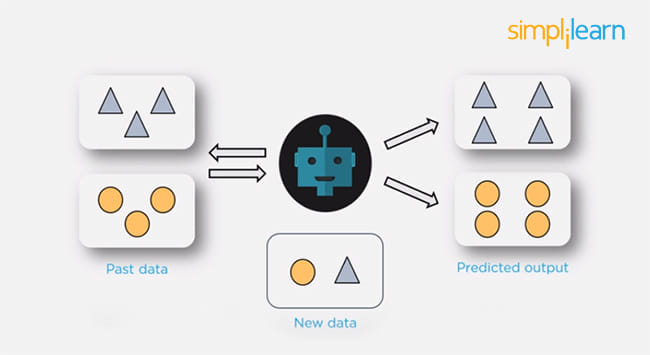#### Unsupervised Learning

In unsupervised learning, we don't have labeled data. A model can identify patterns, anomalies, and relationships in the input data.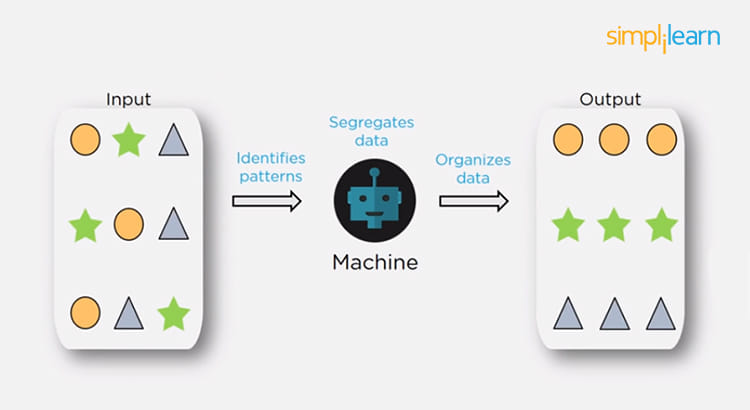#### Reinforcement Learning

Using reinforcement learning, the model can learn based on the rewards it received for its previous action.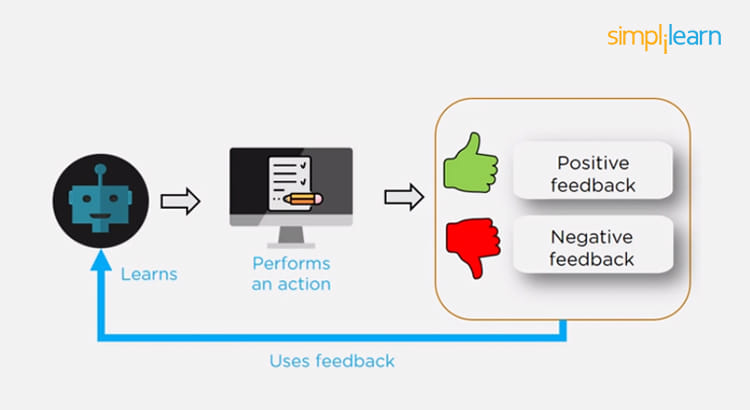Consider an environment where an agent is working. The agent is given a target to achieve. Every time the agent takes some action toward the target, it is given positive feedback. And, if the action taken is going away from the goal, the agent is given negative feedback.

#### 2. What is Overfitting, and How Can You Avoid It?

Overfitting is a situation that occurs when a model learns the training set too well, taking up random fluctuations in the training data as concepts. These impact the model’s ability to generalize and don’t apply to new data.
When a model is given the training data, it shows 100 percent accuracy—technically a slight loss. But, when we use the test data, there may be an error and low efficiency. This condition is known as overfitting.
There are multiple ways of avoiding overfitting, such as:
• Regularization. It involves a cost term for the features involved with the objective function
• Making a simple model. With lesser variables and parameters, the variance can be reduced
• Cross-validation methods like k-folds can also be used
• If some model parameters are likely to cause overfitting, techniques for regularization like LASSO can be used that penalize these parameters

#### 3. What is ‘training Set’ and ‘test Set’ in a Machine Learning Model? How Much Data Will You Allocate for Your Training, Validation, and Test Sets?

There is a three-step process followed to create a model:
1. 1.
Train the model
2. 2.
Test the model
3. 3.
Deploy the model
 Training Set Test Set The training set is examples given to the model to analyze and learn70% of the total data is typically taken as the training datasetThis is labeled data used to train the model The test set is used to test the accuracy of the hypothesis generated by the modelRemaining 30% is taken as testing datasetWe test without labeled data and then verify results with labels
Consider a case where you have labeled data for 1,000 records. One way to train the model is to expose all 1,000 records during the training process. Then you take a small set of the same data to test the model, which would give good results in this case.
But, this is not an accurate way of testing. So, we set aside a portion of that data called the ‘test set’ before starting the training process. The remaining data is called the ‘training set’ that we use for training the model. The training set passes through the model multiple times until the accuracy is high, and errors are minimized.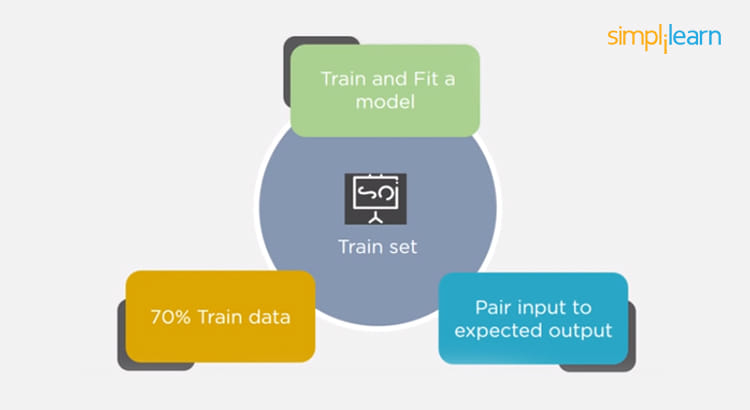Now, we pass the test data to check if the model can accurately predict the values and determine if training is effective. If you get errors, you either need to change your model or retrain it with more data.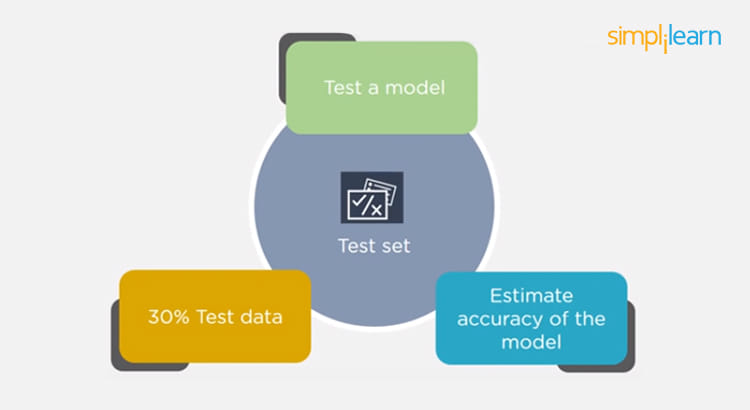Regarding the question of how to split the data into a training set and test set, there is no fixed rule, and the ratio can vary based on individual preferences.

#### 4. How Do You Handle Missing or Corrupted Data in a Dataset?

One of the easiest ways to handle missing or corrupted data is to drop those rows or columns or replace them entirely with some other value.
There are two useful methods in Pandas:
• IsNull() and dropna() will help to find the columns/rows with missing data and drop them
• Fillna() will replace the wrong values with a placeholder value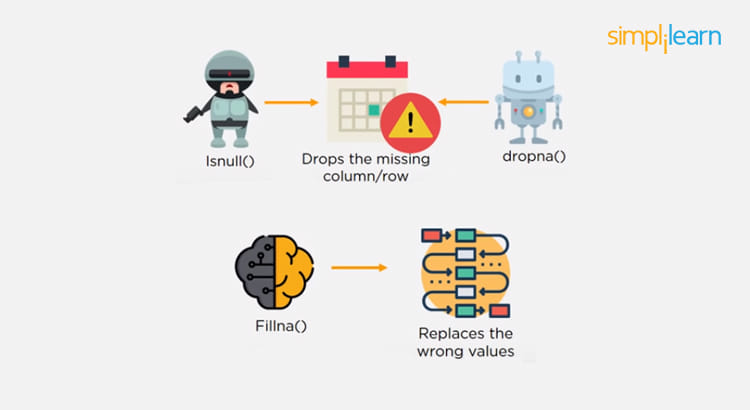#### 5. How Can You Choose a Classifier Based on a Training Set Data Size?

When the training set is small, a model that has a right bias and low variance seems to work better because they are less likely to overfit.
For example, Naive Bayes works best when the training set is large. Models with low bias and high variance tend to perform better as they work fine with complex relationships.

#### 6. Explain the Confusion Matrix with Respect to Machine Learning Algorithms.

A confusion matrix (or error matrix) is a specific table that is used to measure the performance of an algorithm. It is mostly used in supervised learning; in unsupervised learning, it’s called the matching matrix.
The confusion matrix has two parameters:
• Actual
• Predicted
It also has identical sets of features in both of these dimensions.
Consider a confusion matrix (binary matrix) shown below: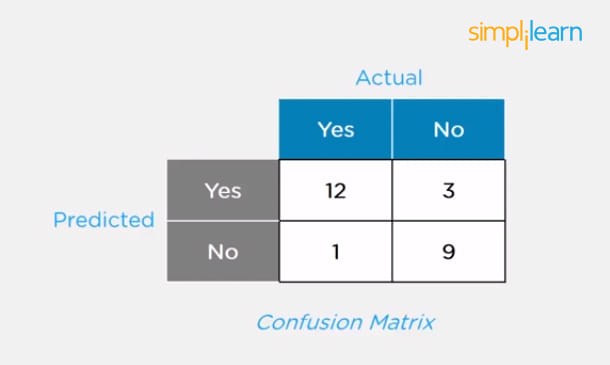Here,
For actual values:
Total Yes = 12+1 = 13
Total No = 3+9 = 12
Similarly, for predicted values:
Total Yes = 12+3 = 15
Total No = 1+9 = 10
For a model to be accurate, the values across the diagonals should be high. The total sum of all the values in the matrix equals the total observations in the test data set.
For the above matrix, total observations = 12+3+1+9 = 25
Now, accuracy = sum of the values across the diagonal / total dataset
= (12+9) / 25
= 21 / 25
= 84%

#### 7. What Is a False Positive and False Negative and How Are They Significant?

False positives are those cases which wrongly get classified as True but are False.
False negatives are those cases which wrongly get classified as False but are True.
In the term ‘False Positive,’ the word ‘Positive’ refers to the ‘Yes’ row of the predicted value in the confusion matrix. The complete term indicates that the system has predicted it as a positive, but the actual value is negative.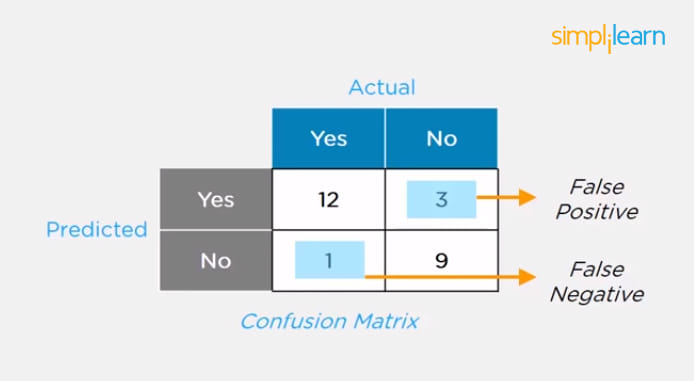So, looking at the confusion matrix, we get:
False-positive = 3
True positive = 12
Similarly, in the term ‘False Negative,’ the word ‘Negative’ refers to the ‘No’ row of the predicted value in the confusion matrix. And the complete term indicates that the system has predicted it as negative, but the actual value is positive.
So, looking at the confusion matrix, we get:
False Negative = 1
True Negative = 9

#### 8. What Are the Three Stages of Building a Model in Machine Learning?

The three stages of building a machine learning model are:
• Model Building
Choose a suitable algorithm for the model and train it according to the requirement
• Model Testing
Check the accuracy of the model through the test data
• Applying the Model
Make the required changes after testing and use the final model for real-time projects
Here, it’s important to remember that once in a while, the model needs to be checked to make sure it’s working correctly. It should be modified to make sure that it is up-to-date.

#### 9. What is Deep Learning?

Deep learning is a subset of machine learning that involves systems that think and learn like humans using artificial neural networks. The term ‘deep’ comes from the fact that you can have several layers of neural networks.
One of the primary differences between machine learning and deep learning is that feature engineering is done manually in machine learning. In the case of deep learning, the model consisting of neural networks will automatically determine which features to use (and which not to use).

#### 10. What Are the Differences Between Machine Learning and Deep Learning?

 Machine Learning Deep Learning Enables machines to take decisions on their own, based on past dataIt needs only a small amount of data for trainingWorks well on the low-end system, so you don't need large machines Most features need to be identified in advance and manually codedThe problem is divided into two parts and solved individually and then combined Enables machines to take decisions with the help of artificial neural networksIt needs a large amount of training data Needs high-end machines because it requires a lot of computing power The machine learns the features from the data it is providedThe problem is solved in an end-to-end manner

#### 11. What Are the Applications of Supervised Machine Learning in Modern Businesses?

Applications of supervised machine learning include:
• Email Spam Detection
Here we train the model using historical data that consists of emails categorized as spam or not spam. This labeled information is fed as input to the model.
• Healthcare Diagnosis
By providing images regarding a disease, a model can be trained to detect if a person is suffering from the disease or not.
• Sentiment Analysis
This refers to the process of using algorithms to mine documents and determine whether they’re positive, neutral, or negative in sentiment.
• Fraud Detection
Training the model to identify suspicious patterns, we can detect instances of possible fraud.

#### 12. What is Semi-supervised Machine Learning?

Supervised learning uses data that is completely labeled, whereas unsupervised learning uses no training data.
In the case of semi-supervised learning, the training data contains a small amount of labeled data and a large amount of unlabeled data.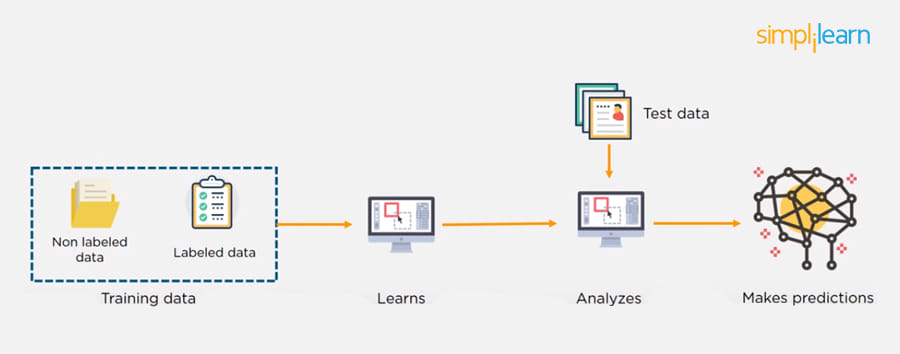#### 13. What Are Unsupervised Machine Learning Techniques?

There are two techniques used in unsupervised learning: clustering and association.

#### Clustering

Clustering problems involve data to be divided into subsets. These subsets, also called clusters, contain data that are similar to each other. Different clusters reveal different details about the objects, unlike classification or regression.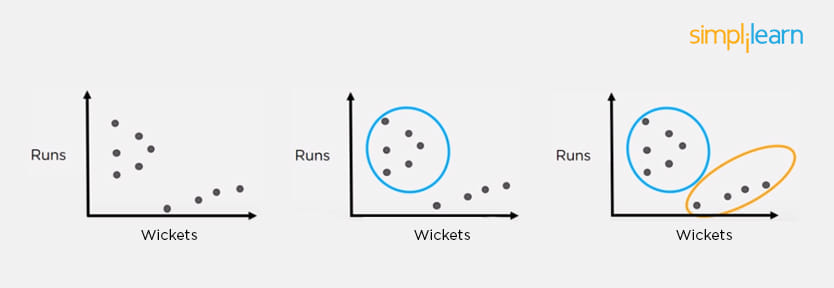#### Association

In an association problem, we identify patterns of associations between different variables or items.
For example, an e-commerce website can suggest other items for you to buy, based on the prior purchases that you have made, spending habits, items in your wishlist, other customers’ purchase habits, and so on.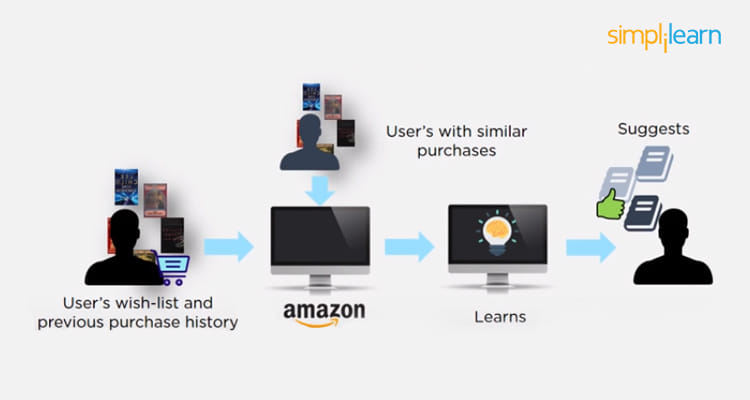#### 14. What is the Difference Between Supervised and Unsupervised Machine Learning?

• Supervised learning - This model learns from the labeled data and makes a future prediction as output
• Unsupervised learning - This model uses unlabeled input data and allows the algorithm to act on that information without guidance.

#### 15. What is the Difference Between Inductive Machine Learning and Deductive Machine Learning?

 Inductive Learning Deductive Learning It observes instances based on defined principles to draw a conclusionExample: Explaining to a child to keep away from the fire by showing a video where fire causes damage It concludes experiencesExample: Allow the child to play with fire. If he or she gets burned, they will learn that it is dangerous and will refrain from making the same mistake again

#### 16. Compare K-means and KNN Algorithms.

 K-means KNN ​K-Means is unsupervisedK-Means is a clustering algorithmThe points in each cluster are similar to each other, and each cluster is different from its neighboring clusters ​KNN is supervised in natureKNN is a classification algorithmIt classifies an unlabeled observation based on its K (can be any number) surrounding neighbors

#### 17. What Is ‘naive’ in the Naive Bayes Classifier?

The classifier is called ‘naive’ because it makes assumptions that may or may not turn out to be correct.
The algorithm assumes that the presence of one feature of a class is not related to the presence of any other feature (absolute independence of features), given the class variable.
For instance, a fruit may be considered to be a cherry if it is red in color and round in shape, regardless of other features. This assumption may or may not be right (as an apple also matches the description).

#### 18. Explain How a System Can Play a Game of Chess Using Reinforcement Learning.

Reinforcement learning has an environment and an agent. The agent performs some actions to achieve a specific goal. Every time the agent performs a task that is taking it towards the goal, it is rewarded. And, every time it takes a step which goes against that goal or in reverse direction, it is penalized.
Earlier, chess programs had to determine the best moves after much research on numerous factors. Building a machine designed to play such games would require many rules to be specified.
With reinforced learning, we don’t have to deal with this problem as the learning agent learns by playing the game. It will make a move (decision), check if it’s the right move (feedback), and keep the outcomes in memory for the next step it takes (learning). There is a reward for every correct decision the system takes and punishment for the wrong one.

#### 19. How Will You Know Which Machine Learning Algorithm to Choose for Your Classification Problem?

While there is no fixed rule to choose an algorithm for a classification problem, you can follow these guidelines:
• If accuracy is a concern, test different algorithms and cross-validate them
• If the training dataset is small, use models that have low variance and high bias
• If the training dataset is large, use models that have high variance and little bias

#### 20. How is Amazon Able to Recommend Other Things to Buy? How Does the Recommendation Engine Work?

Once a user buys something from Amazon, Amazon stores that purchase data for future reference and finds products that are most likely also to be bought, it is possible because of the Association algorithm, which can identify patterns in a given dataset.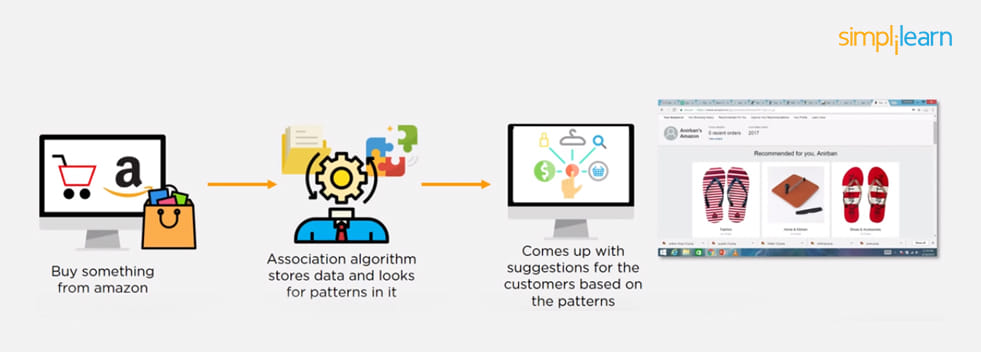#### 21. When Will You Use Classification over Regression?

Classification is used when your target is categorical, while regression is used when your target variable is continuous. Both classification and regression belong to the category of supervised machine learning algorithms.
Examples of classification problems include:
• Predicting yes or no
• Estimating gender
• Breed of an animal
• Type of color
Examples of regression problems include:
• Estimating sales and price of a product
• Predicting the score of a team
• Predicting the amount of rainfall

#### 22. How Do You Design an Email Spam Filter?

Building a spam filter involves the following process:
• The email spam filter will be fed with thousands of emails
• Each of these emails already has a label: ‘spam’ or ‘not spam.’
• The supervised machine learning algorithm will then determine which type of emails are being marked as spam based on spam words like the lottery, free offer, no money, full refund, etc.
• The next time an email is about to hit your inbox, the spam filter will use statistical analysis and algorithms like Decision Trees and SVM to determine how likely the email is spam
• If the likelihood is high, it will label it as spam, and the email won’t hit your inbox
• Based on the accuracy of each model, we will use the algorithm with the highest accuracy after testing all the models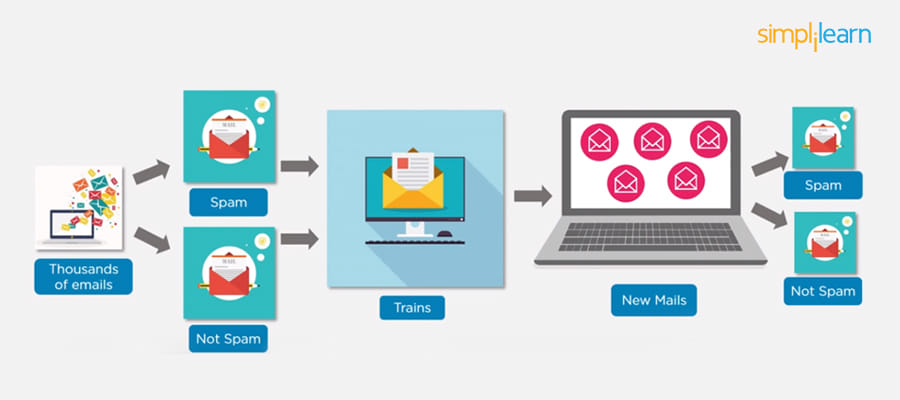#### 23. What is a Random Forest?

A ‘random forest’ is a supervised machine learning algorithm that is generally used for classification problems. It operates by constructing multiple decision trees during the training phase. The random forest chooses the decision of the majority of the trees as the final decision.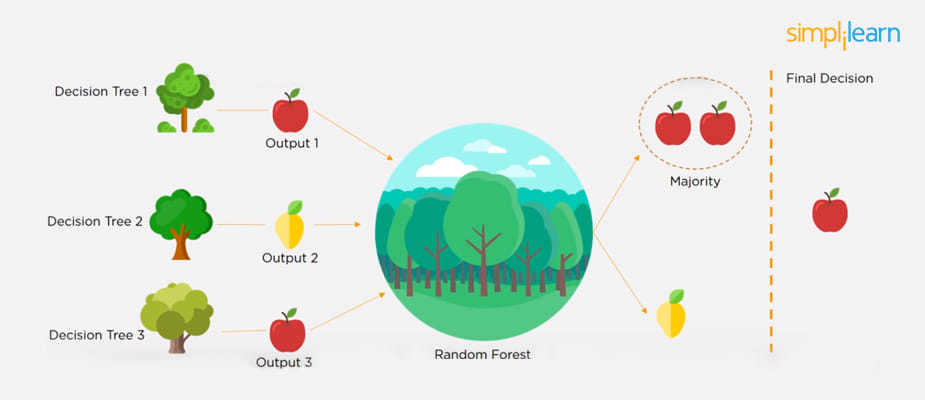#### 24. Considering a Long List of Machine Learning Algorithms, given a Data Set, How Do You Decide Which One to Use?

There is no master algorithm for all situations. Choosing an algorithm depends on the following questions:
• How much data do you have, and is it continuous or categorical?
• Is the problem related to classification, association, clustering, or regression?
• Predefined variables (labeled), unlabeled, or mix?
• What is the goal?
Based on the above questions, the following algorithms can be used: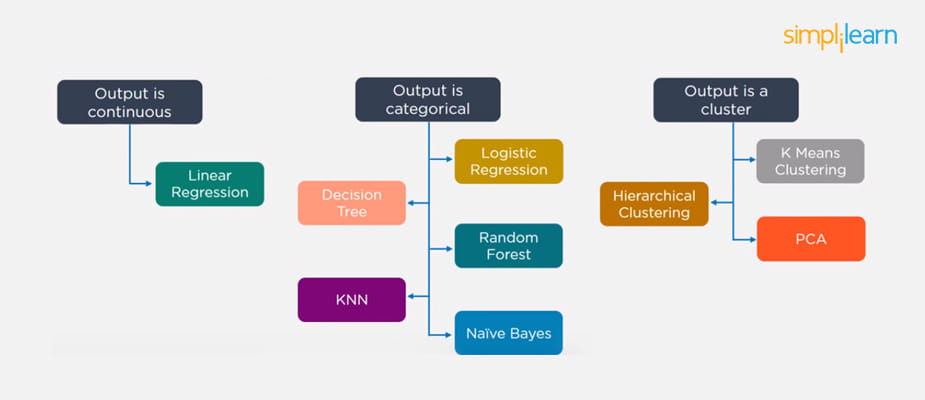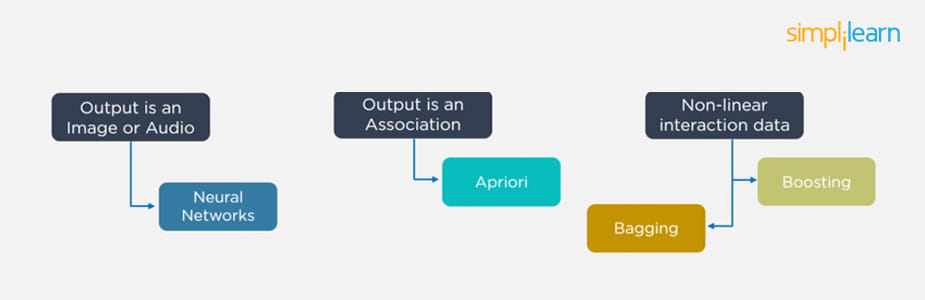#### Bias

Bias in a machine learning model occurs when the predicted values are further from the actual values. Low bias indicates a model where the prediction values are very close to the actual ones.
Underfitting: High bias can cause an algorithm to miss the relevant relations between features and target outputs.

#### Variance

Variance refers to the amount the target model will change when trained with different training data. For a good model, the variance should be minimized.
Overfitting: High variance can cause an algorithm to model the random noise in the training data rather than the intended outputs.

#### 26. What is the Trade-off Between Bias and Variance?

The bias-variance decomposition essentially decomposes the learning error from any algorithm by adding the bias, variance, and a bit of irreducible error due to noise in the underlying dataset.
Necessarily, if you make the model more complex and add more variables, you’ll lose bias but gain variance. To get the optimally-reduced amount of error, you’ll have to trade off bias and variance. Neither high bias nor high variance is desired.
High bias and low variance algorithms train models that are consistent, but inaccurate on average.
High variance and low bias algorithms train models that are accurate but inconsistent.

#### Precision

Precision is the ratio of several events you can correctly recall to the total number of events you recall (mix of correct and wrong recalls).
Precision = (True Positive) / (True Positive + False Positive)

#### Recall

A recall is the ratio of a number of events you can recall the number of total events.
Recall = (True Positive) / (True Positive + False Negative)

#### 28. What is Decision Tree Classification?

A decision tree builds classification (or regression) models as a tree structure, with datasets broken up into ever-smaller subsets while developing the decision tree, literally in a tree-like way with branches and nodes. Decision trees can handle both categorical and numerical data.

#### 29. What is Pruning in Decision Trees, and How Is It Done?

Pruning is a technique in machine learning that reduces the size of decision trees. It reduces the complexity of the final classifier, and hence improves predictive accuracy by the reduction of overfitting.
Pruning can occur in:
• Top-down fashion. It will traverse nodes and trim subtrees starting at the root
• Bottom-up fashion. It will begin at the leaf nodes
There is a popular pruning algorithm called reduced error pruning, in which:
• Starting at the leaves, each node is replaced with its most popular class
• If the prediction accuracy is not affected, the change is kept
• There is an advantage of simplicity and speed

#### 30. Briefly Explain Logistic Regression.

Logistic regression is a classification algorithm used to predict a binary outcome for a given set of independent variables.
The output of logistic regression is either a 0 or 1 with a threshold value of generally 0.5. Any value above 0.5 is considered as 1, and any point below 0.5 is considered as 0.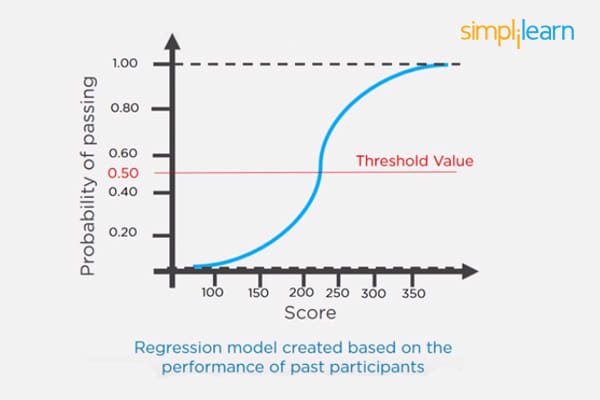#### 31. Explain the K Nearest Neighbor Algorithm.

K nearest neighbor algorithm is a classification algorithm that works in a way that a new data point is assigned to a neighboring group to which it is most similar.
In K nearest neighbors, K can be an integer greater than 1. So, for every new data point, we want to classify, we compute to which neighboring group it is closest.
Let us classify an object using the following example. Consider there are three clusters:
• Football
• Tennis ball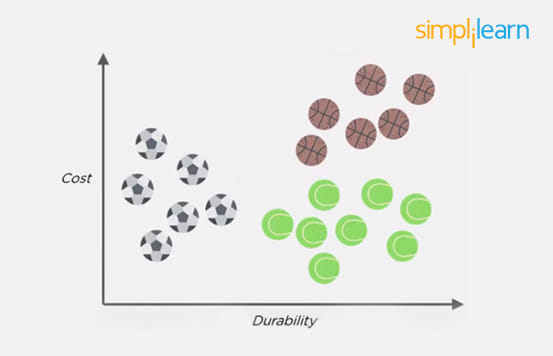Let the new data point to be classified is a black ball. We use KNN to classify it. Assume K = 5 (initially).
Next, we find the K (five) nearest data points, as shown.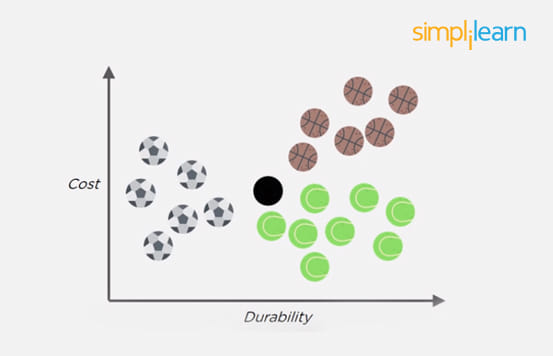Observe that all five selected points do not belong to the same cluster. There are three tennis balls and one each of basketball and football.
When multiple classes are involved, we prefer the majority. Here the majority is with the tennis ball, so the new data point is assigned to this cluster.

#### 32. What is a Recommendation System?

Anyone who has used Spotify or shopped at Amazon will recognize a recommendation system: It’s an information filtering system that predicts what a user might want to hear or see based on choice patterns provided by the user.

#### 33. What is Kernel SVM?

Kernel SVM is the abbreviated version of the kernel support vector machine. Kernel methods are a class of algorithms for pattern analysis, and the most common one is the kernel SVM.

#### 34. What Are Some Methods of Reducing Dimensionality?

You can reduce dimensionality by combining features with feature engineering, removing collinear features, or using algorithmic dimensionality reduction.
Now that you have gone through these machine learning interview questions, you must have got an idea of your strengths and weaknesses in this domain.

## Question Set 3

#### 1. What are the types of Machine Learning?

In all the ML Interview Questions that we would be going to discuss, this is one of the most basic question.
So, basically, there are three types of Machine Learning techniques:
Supervised Learning: In this type of the Machine Learning technique, machines learn under the supervision of labeled data. There is a training dataset on which the machine is trained, and it gives the output according to its training.
Unsupervised Learning: Unlike supervised learning, it has unlabeled data. So, there is no supervision under which it works on the data. Basically, unsupervised learning tries to identify patterns in data and make clusters of similar entities. After that, when a new input data is fed into the model, it does not identify the entity; rather, it puts the entity in a cluster of similar objects.
Reinforcement Learning: Reinforcement learning includes models that learn and traverse to find the best possible move. The algorithms for reinforcement learning are constructed in a way that they try to find the best possible suite of action on the basis of the reward and punishment theory.

#### 2. Differentiate between classification and regression in Machine Learning.

In Machine Learning, there are various types of prediction problems based on supervised and unsupervised learning. These are classification, regression, clustering, and association. Here, we will discuss about classification and regression.
Classification: In classification, we try to create a Machine Learning model that assists us in differentiating data into separate categories. The data is labeled and categorized based on the input parameters.
For example, imagine that we want to make predictions on the churning out customers for a particular product based on some data recorded. Either the customers will churn out or they will not. So, the labels for this would be ‘Yes’ and ‘No.’
Regression: It is the process of creating a model for distinguishing data into continuous real values, instead of using classes or discrete values. It can also identify the distribution movement depending on the historical data. It is used for predicting the occurrence of an event depending on the degree of association of variables.
For example, the prediction of weather condition depends on factors such as temperature, air pressure, solar radiation, elevation of the area, and distance from sea. The relation between these factors assists us in predicting the weather condition.

#### 3. What is Linear Regression?

Linear Regression is a supervised Machine Learning algorithm. It is used to find the linear relationship between the dependent and the independent variables for predictive analysis.
The equation for Linear Regression:
Y = A + BX
where:
• X is the input or the independent variable
• Y is the output or the dependent variable
• a is the intercept and b is the coefficient of X
Below is the best fit line that shows the data of weight (Y or the dependent variable) and height (X or the independent variable) of 21-years-old candidates scattered over the plot. This straight line shows the best linear relationship that would help in predicting the weight of candidates according to their height.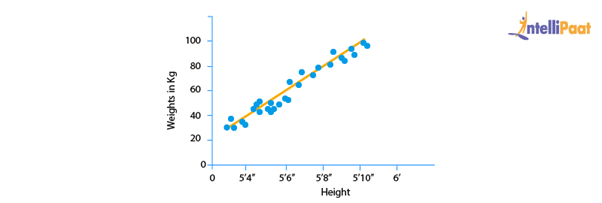To get this best fit line, we will try to find the best values of a and b. By adjusting the values of a and b, we will try to reduce errors in the prediction of Y.
This is how linear regression helps in finding the linear relationship and predicting the output.

#### 4. How will you determine the Machine Learning algorithm that is suitable for your problem?

To identify the Machine Learning algorithm for our problem, we should follow the below steps:
Step 1: Problem Classification: Classification of the problem depends on the classification of input and output:
• Classifying the input: Classification of the input depends on whether we have data labeled (supervised learning) or unlabeled (unsupervised learning), or whether we have to create a model that interacts with the environment and improves itself (reinforcement learning).
• Classifying the output: If we want the output of our model as a class, then we need to use some classification techniques.
If it is giving the output as a number, then we must use regression techniques and, if the output is a different cluster of inputs, then we should use clustering techniques.
Step 2: Checking the algorithms in hand: After classifying the problem, we have to look for the available algorithms that can be deployed for solving the classified problem.
Step 3: Implementing the algorithms: If there are multiple algorithms available, then we will implement each one of them, one by one. Finally, we would select the algorithm that gives the best performance.

#### 5. What are Bias and Variance?

• Bias is the difference between the average prediction of our model and the correct value. If the bias value is high, then the prediction of the model is not accurate. Hence, the bias value should be as low as possible to make the desired predictions.
• Variance is the number that gives the difference of prediction over a training set and the anticipated value of other training sets. High variance may lead to large fluctuation in the output. Therefore, the model’s output should have low variance.
The below diagram shows the bias–variance trade off: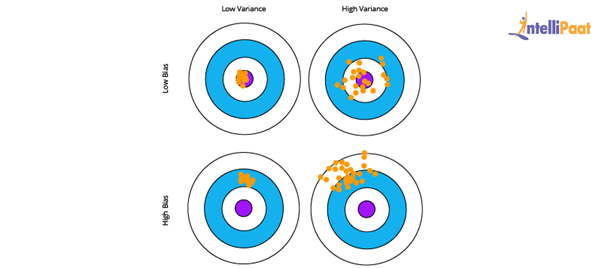Here, the desired result is the blue circle at the center. If we get off from the blue section, then the prediction goes wrong.

#### 6. What is Variance Inflation Factor?

Variance Inflation Factor (VIF) is the estimate of the volume of multicollinearity in a collection of many regression variables.
VIF = Variance of the model / Variance of the model with a single independent variable
We have to calculate this ratio for every independent variable. If VIF is high, then it shows the high collinearity of the independent variables.

#### 7. Explain false negative, false positive, true negative, and true positive with a simple example.

True Positive (TP): When the Machine Learning model correctly predicts the condition, it is said to have a True Positive value.
True Negative (TN): When the Machine Learning model correctly predicts the negative condition or class, then it is said to have a True Negative value.
False Positive (FP): When the Machine Learning model incorrectly predicts a negative class or condition, then it is said to have a False Positive value.
False Negative (FN): When the Machine Learning model incorrectly predicts a positive class or condition, then it is said to have a False Negative value.

#### 8. What is a Confusion Matrix?

Confusion matrix is used to explain a model’s performance and gives the summary of predictions on the classification problems. It assists in identifying the uncertainty between classes.
A confusion matrix gives the count of correct and incorrect values and also the error types.Accuracy of the model:Accuracy of the model
For example, consider this confusion matrix. It consists of values as True Positive, True Negative, False Positive, and False Negative for a classification model. Now, the accuracy of the model can be calculated as follows:What is a Confusion Matrix
Thus, in our example:
Accuracy = (200 + 50) / (200 + 50 + 10 + 60) = 0.78
This means that the model’s accuracy is 0.78, corresponding to its True Positive, True Negative, False Positive, and False Negative values.

#### 9. What do you understand by Type I and Type II errors?

Type I Error: Type I error (False Positive) is an error where the outcome of a test shows the non-acceptance of a true condition.
For example, a cricket match is going on and, when a batsman is not out, the umpire declares that he is out. This is a false positive condition. Here, the test does not accept the true condition that the batsman is not out.
Type II Error: Type II error (False Negative) is an error where the outcome of a test shows the acceptance of a false condition.
For example, the CT scan of a person shows that he is not having a disease but, in reality, he is having it. Here, the test accepts the false condition that the person is not having the disease.

#### 10. When should you use classification over regression?

Both classification and regression are associated with prediction. Classification involves the identification of values or entities that lie in a specific group. The regression method, on the other hand, entails predicting a response value from a consecutive set of outcomes.
The classification method is chosen over regression when the output of the model needs to yield the belongingness of data points in a dataset to a particular category.
For example, we have some names of bikes and cars. We would not be interested in finding how these names are correlated to bikes and cars. Rather, we would check whether each name belongs to the bike category or to the car category.

#### 11. Explain Logistic Regression.

Logistic regression is the proper regression analysis used when the dependent variable is categorical or binary. Like all regression analyses, logistic regression is a technique for predictive analysis. Logistic regression is used to explain data and the relationship between one dependent binary variable and one or more independent variables. Also, it is employed to predict the probability of a categorical dependent variable.
We can use logistic regression in the following scenarios:
• To predict whether a citizen is a Senior Citizen (1) or not (0)
• To check whether a person is having a disease (Yes) or not (No)
There are three types of logistic regression:
• Binary Logistic Regression: In this, there are only two outcomes possible.
Example: To predict whether it will rain (1) or not (0)
• Multinomial Logistic Regression: In this, the output consists of three or more unordered categories.
Example: Prediction on the regional languages (Kannada, Telugu, Marathi, etc.)
• Ordinal Logistic Regression: In ordinal logistic regression, the output consists of three or more ordered categories.
Example: Rating an Android application from 1 to 5 stars.

#### 12. Imagine, you are given a dataset consisting of variables having more than 30% missing values. Let’s say, out of 50 variables, 8 variables have missing values, which is higher than 30%. How will you deal with them?

To deal with the missing values, we will do the following:
• We will specify a different class for the missing values.
• Now, we will check the distribution of values, and we would hold those missing values that are defining a pattern.
• Then, we will charge these into a yet another class, while eliminating others.

#### 13. How do you handle the missing or corrupted data in a dataset?

In Python Pandas, there are two methods that are very useful. We can use these two methods to locate the lost or corrupted data and discard those values:
• isNull(): For detecting the missing values, we can use the isNull() method.
• dropna(): For removing the columns/rows with null values, we can use the dropna() method.
Also, we can use fillna() to fill the void values with a placeholder value.

#### 14. Explain Principal Component Analysis (PCA).

Firstly, this is one of the most important Machine Learning Interview Questions.
In the real world, we deal with multi-dimensional data. Thus, data visualization and computation become more challenging with the increase in dimensions. In such a scenario, we might have to reduce the dimensions to analyze and visualize the data easily. We do this by:
• Removing irrelevant dimensions
• Keeping only the most relevant dimensions
This is where we use Principal Component Analysis (PCA).
Finding a fresh collection of uncorrelated dimensions (orthogonal) and ranking them on the basis of variance are the goals of Principal Component Analysis.
The Mechanism of PCA:
• Compute the covariance matrix for data objects
• Compute the Eigen vectors and the Eigen values in a descending order
• To get the new dimensions, select the initial N Eigen vectors
• Finally, change the initial n-dimensional data objects into N-dimensions
Example: Below are the two graphs showing data points (objects) and two directions: one is ‘green’ and the other is ‘yellow.’ We got the Graph 2 by rotating the Graph 1 so that the x-axis and y-axis represent the ‘green’ and ‘yellow’ directions, respectively.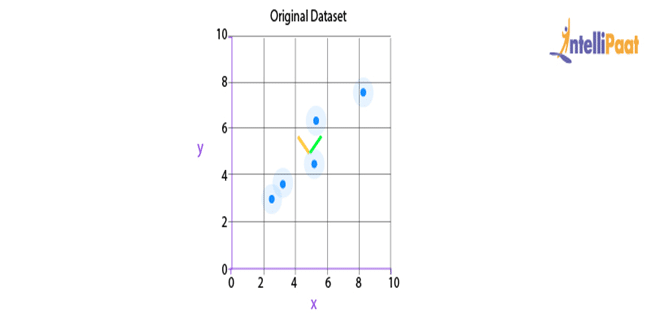Explain Principal Component Analysis (PCA)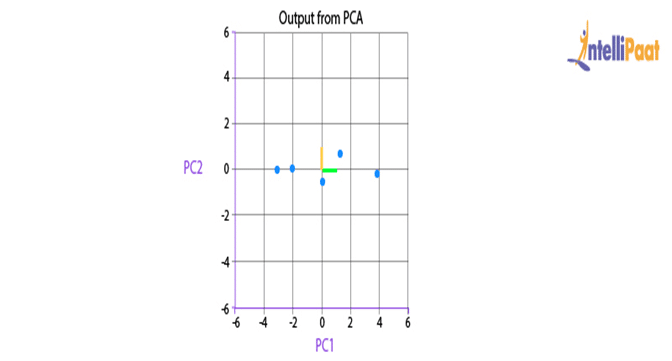Output from PCA
After the rotation of the data points, we can infer that the green direction (x-axis) gives us the line that best fits the data points.
Here, we are representing 2-dimensional data. But in real-life, the data would be multi-dimensional and complex. So, after recognizing the importance of each direction, we can reduce the area of dimensional analysis by cutting off the less-significant ‘directions.’
Now, we will look into another important Machine Learning Interview Question on PCA.

#### 15. Why rotation is required in PCA? What will happen if you don’t rotate the components?

Rotation is a significant step in PCA as it maximizes the separation within the variance obtained by components. Due to this, the interpretation of components becomes easier.
The motive behind doing PCA is to choose fewer components that can explain the greatest variance in a dataset. When rotation is performed, the original coordinates of the points get changed. However, there is no change in the relative position of the components.
If the components are not rotated, then we need more extended components to describe the variance.

#### 16. We know that one hot encoding increases the dimensionality of a dataset, but label encoding doesn’t. How?

When we use one hot encoding, there is an increase in the dimensionality of a dataset. The reason for the increase in dimensionality is that, for every class in the categorical variables, it forms a different variable.
Example: Suppose, there is a variable ‘Color.’ It has three sub-levels as Yellow, Purple, and Orange. So, one hot encoding ‘Color’ will create three different variables as Color.Yellow, Color.Porple, and Color.Orange.
In label encoding, the sub-classes of a certain variable get the value as 0 and 1. So, we use label encoding only for binary variables.
This is the reason that one hot encoding increases the dimensionality of data and label encoding does not.

#### 17. How can you avoid overfitting?

Overfitting happens when a machine has an inadequate dataset and it tries to learn from it. So, overfitting is inversely proportional to the amount of data.
For small databases, we can bypass overfitting by the cross-validation method. In this approach, we will divide the dataset into two sections. These two sections will comprise testing and training sets. To train the model, we will use the training dataset and, for testing the model for new inputs, we will use the testing dataset.
This is how we can avoid overfitting.

#### 18. Why do we need a validation set and a test set?

We split the data into three different categories while creating a model:
1. 1.
Training set: We use the training set for building the model and adjusting the model’s variables. But, we cannot rely on the correctness of the model build on top of the training set. The model might give incorrect outputs on feeding new inputs.
2. 2.
Validation set: We use a validation set to look into the model’s response on top of the samples that don’t exist in the training dataset. Then, we will tune hyperparameters on the basis of the estimated benchmark of the validation data.
When we are evaluating the model’s response using the validation set, we are indirectly training the model with the validation set. This may lead to the overfitting of the model to specific data. So, this model won’t be strong enough to give the desired response to the real-world data.
1. 1.
Test set: The test dataset is the subset of the actual dataset, which is not yet used to train the model. The model is unaware of this dataset. So, by using the test dataset, we can compute the response of the created model on hidden data. We evaluate the model’s performance on the basis of the test dataset.
Note: We always expose the model to the test dataset after tuning the hyperparameters on top of the validation set.
As we know, the evaluation of the model on the basis of the validation set would not be enough. Thus, we use a test set for computing the efficiency of the model.

#### 19. What is a Decision Tree?

A decision tree is used to explain the sequence of actions that must be performed to get the desired output. It is a hierarchical diagram that shows the actions.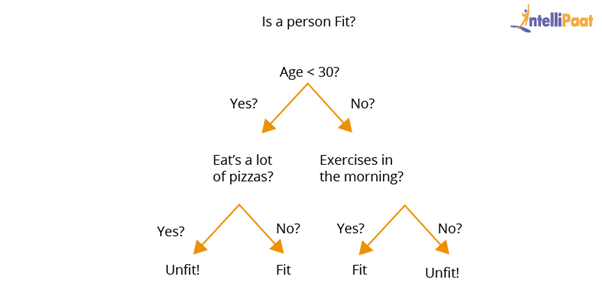What is a Decision Tree
We can create an algorithm for a decision tree on the basis of the hierarchy of actions that we have set.
In the above decision tree diagram, we have made a sequence of actions for driving a vehicle with/without a license.

#### 20. Explain the difference between KNN and K-means Clustering.

K-nearest neighbors: It is a supervised Machine Learning algorithm. In KNN, we give the identified (labeled) data to the model. Then, the model matches the points based on the distance from the closest points.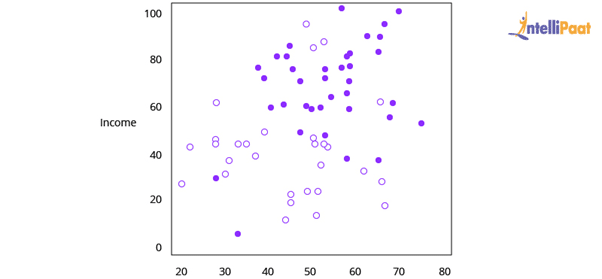K-nearest neighbors
K-means clustering: It is an unsupervised Machine Learning algorithm. In this, we give the unidentified (unlabeled) data to the model. Then, the algorithm creates batches of points based on the average of the distances between distinct points.K-means clustering

#### 21. What is Dimensionality Reduction?

In the real world, we build Machine Learning models on top of features and parameters. These features can be multi-dimensional and large in number. Sometimes, the features may be irrelevant and it becomes a difficult task to visualize them.
Here, we use dimensionality reduction to cut down the irrelevant and redundant features with the help of principal variables. These principal variables are the subgroup of the parent variables that conserve the feature of the parent variables.

#### 22. Both being tree-based algorithms, how is Random Forest different from Gradient Boosting Algorithm (GBM)?

The main difference between a random forest and GBM is the use of techniques. Random forest advances predictions using a technique called ‘bagging.’ On the other hand, GBM advances predictions with the help of a technique called ‘boosting.’
• Bagging: In bagging, we apply arbitrary sampling and we divide the dataset into N After that, we build a model by employing a single training algorithm. Following, we combine the final predictions by polling. Bagging helps increase the efficiency of the model by decreasing the variance to eschew overfitting.
• Boosting: In boosting, the algorithm tries to review and correct the inadmissible predictions at the initial iteration. After that, the algorithm’s sequence of iterations for correction continues until we get the desired prediction. Boosting assists in reducing bias and variance, both, for making the weak learners strong.

#### 23. Suppose, you found that your model is suffering from high variance. Which algorithm do you think could handle this situation and why?

Handling High Variance
• For handling issues of high variance, we should use the bagging algorithm.
• Bagging algorithm would split data into sub-groups with replicated sampling of random data.
• Once the algorithm splits the data, we use random data to create rules using a particular training algorithm.
• After that, we use polling for combining the predictions of the model.